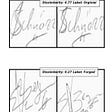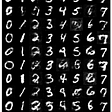# Tensorflow 數據模型 — — 張量

`a = tf.constant([1.0, 2.0], name=’a’)b = tf.constant([2.0, 3.0], name=’b’)result = tf.add(a, b, name=’add’)print(result)`
`Tensor(“add_1:0”, shape=(2,), dtype=float32)`
`a = tf.constant([1, 2], name=’a’)b = tf.constant([2.0, 3.0], name=’b’)result = tf.add(a, b, name=’add’)`
`ValueError: Tensor conversion requested dtype int32 for Tensor with dtype float32: ‘Tensor(“b_2:0”, shape=(2,), dtype=float32)’`
`result.get_shape()`
`TensorShape([Dimension(2)])`

# TensorFlow 運行模型 — — 會話()

`sess = tf.Session()print(sess.run(result))sess.close()`
`[3. 5.]`
`with tf.Session() as sess:    print(sess.run(result))`
`[3. 5.]`
`with tf.Session() as sess:    print(sess.run(result))    print(result.eval())`

## tf.constant

`node1 = tf.constant(3.0, tf.float32)node2 = tf.constant(4)tf.add(node1, node2)`
`Input ‘y’ of ‘Add’ Op has type int32 that does not match type float32 of argument ‘x’.`

## tf.placeholder

`# 建構計算圖a = tf.placeholder(tf.float32)b = tf.placeholder(tf.float32)add_node = a + b`
`print(add_node)# 運行計算圖print(sess.run(add_node, feed_dict={a: 1, b: 3}))print(sess.run(add_node, feed_dict={a: [1, 2], b: [3, 4]}))`
`<tf.Tensor ‘add:0’ shape=<unknown> dtype=float32>4.0[4. 6.]`
`add_and_triple = add_node * 3add_and_triple# 運行計算圖print(sess.run(add_and_triple, feed_dict={a: [1, 2], b: [3, 4]}))`
`<tf.Tensor ‘mul:0’ shape=<unknown> dtype=float32>[12. 18.]`

## tf.Variable

`n1 = tf.Variable(3.0, tf.float32)`
`init = tf.global_variables_initializer()sess.run(init)`
`sess.run(n1)`
`3.0`
`W = tf.Variable([1., 3, 5.])b = tf.Variable([1.], dtype=tf.float32)X = tf.placeholder(dtype=tf.float32, shape=(3,))net = W * X + binit = tf.global_variables_initializer()sess.run(init)sess.run(net, feed_dict={X: [1., 2., 3]})`
`array([ 2., 7., 16.], dtype=float32)`

## tf.train

`# optimizeroptimizer = tf.train.GradientDescentOptimizer(learning_rate=0.01)train = optimizer.minimize(loss)# trainingepoch = 1000for _ in range(epoch):    sess.run(train, feed_dict={X: [1., 2., 3.], y: [3., 6., 15.]})`
`W = tf.Variable([1., 3, 5.])b = tf.Variable([1.], dtype=tf.float32)X = tf.placeholder(dtype=tf.float32, shape=(3,))net = W * X + binit = tf.global_variables_initializer()sess.run(init)# labely = tf.placeholder(dtype=tf.float32)# loss functionsquare_errors = tf.square(net-y)loss = tf.reduce_sum(square_errors)# optimizeroptimizer = tf.train.GradientDescentOptimizer(learning_rate=0.01)train = optimizer.minimize(loss)# trainingepoch = 1000for _ in range(epoch):    sess.run(train, feed_dict={X: [1., 2., 3.], y: [3., 6., 15.]})curr_W, curr_b, curr_loss = sess.run([W, b, loss],{X:[ 1 , 2 , 3], y: [3., 6., 15.]}) print ( “W: {} b: {} loss: {}”.format(curr_W, curr_b, curr_loss))`
`W: [1.7294136 2.3647099 4.576473 ] b: [1.2705834] loss: 2.4556356947869062e-11`

--

--

--

## More from Airwaves

Love podcasts or audiobooks? Learn on the go with our new app.## Deploying AI Models on Edge using OpenVino## Handwritten Signature Verification## Introduction to auto encoders## How to build a CGAN for generating MNIST digits in PyTorch